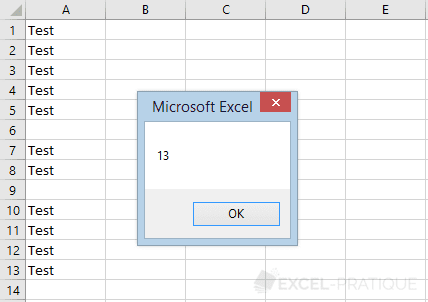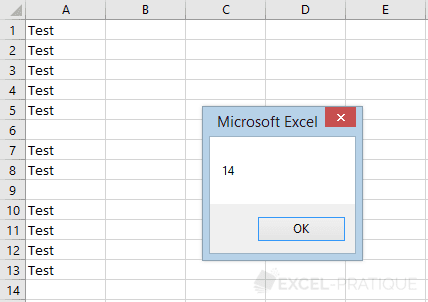# VBA Tip: ﻿Determining the Number of the Last Row

You often need to insert data at the end of a table in an Excel worksheet, and to do this, you need to know the number of the first empty row available in the table.

The number of the last non-empty row in a table is obtained using:

``Cells(Rows.Count, COLUMN).End(xlUp).Row``

## First empty cell in column A

To find out the last non-empty cell in a column, use the code above and replace "COLUMN" with 1:

``````Sub example()

lastRowNum = Cells(Rows.Count, 1).End(xlUp).Row

MsgBox lastRowNum

End Sub``````

This gives you the number of the last non-empty row in column A:To obtain the number of the first empty row, simply add 1 to the result:

``````Sub example()

lastRowNum = Cells(Rows.Count, 1).End(xlUp).Row + 1

MsgBox lastRowNum

End Sub``````

This now gives you the number of the first empty row in column A:To summarize how this works, the search begins at the last row in the worksheet (`Rows.Count`) then works upwards (`xlUp`) until it finds a non-empty cell.

## First non-empty cell using the column letter

If you have a column letter rather than a column number, use Range in place of Cells:

``````Sub example()

lastRowNum = Range("A" & Rows.Count).End(xlUp).Row + 1

MsgBox lastRowNum

End Sub``````